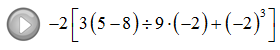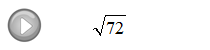## Pages

Showing posts with label algebra 1. Show all posts
Showing posts with label algebra 1. Show all posts

### Chapter 1 Sample Test Questions

Click here for a worksheet containing 20 sample test questions with answers.

Here are a few solved problems:
Word Problem:  Joe’s bill came to \$12.05 before a 7 1/4%  tax was added. What is his total bill after tax is added?

### Order of Operations

When several operations are to be applied within a calculation, we must follow a specific order to ensure a single correct result.

Order of Operations:
1. Perform all calculations within the innermost Parentheses first.
2. Evaluate Exponent expressions.
3. Apply the Multiplication and Division operations from left to right.
4. Lastly, work all Addition and Subtraction operations from left to right.
Caution:  Please do not dismiss the fact that multiplication and division should be worked from left to right.  Many standardized exams will test you on this fact.  The following example illustrates the problem.

Instructional Video: Order of Operations - The Basics

Simplify.

We will see that some of the problems have different looking parentheses { [ ( ) ] }, treat them the same and just remember to perform the innermost parentheses first.  Some problems may involve an absolute value, in which case you will need to apply the innermost absolute value first as you would if it were a parentheses.
To avoid these unnecessary mistakes, work one operation at a time and for each step rewrite everything.  This may seem like a lot of work but it really helps avoid errors.

Simplify.

Video Examples on YouTube:### Exponents and Square Roots

To square a number means to multiply that number times itself.  For example:
The number 5 is called the base and the integer 2 is called the exponent.

[ Video Instruction: Exponential Notation ]

Another way to read the above example is "5 raised to the second power."

Simplify.
It is important to point out the last two solved problems, what is different?

The square root can be thought of as the opposite of squaring a number.  In other words, if we want the square root of 16 the question is "what number squared gives 16?"  Actually there are two answers to this question, 4 and −4 because:
Hence, there is a technical distinction here. When we are asked for the square root of numbers we mean the principal (non-negative) square root.

Simplify.
If A and B are positive and real the following property is useful for simplifying square roots:
Look for the largest perfect square factor and the apply this property as follows:
Video Examples on YouTube: